<![CDATA[Canadian Journal of Mathematics]]>https://www.cambridge.org/core/journals/canadian-journal-of-mathematics/latest-issueCanadian Journal of Mathematics0008414X14964279Cambridge University Press<![CDATA[The Action of a Plane Singular Holomorphic Flow on a Non-invariant Branch]]>

We study the dynamics of a singular holomorphic vector field at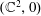. Using the associated flow and its pullback to the blow-up manifold, we provide invariants relating the vector field, a non-invariant analytic branch of curve, and the deformation of this branch by the flow. This leads us to study the conjugacy classes of singular branches under the action of holomorphic flows. In particular, we show that there exists an analytic class that is not complete, meaning that there are two elements of the class that are not analytically conjugated by a local biholomorphism embedded in a one-parameter flow. Our techniques are new and offer an approach dual to the one used classically to study singularities of holomorphic vector fields.

]]>
https://dx.doi.org/10.4153/S0008414X19000221?rft_dat=source%3Ddrssdoi:10.4153/S0008414X19000221The Action of a Plane Singular Holomorphic Flow on a Non-invariant Branch83586610.4153/S0008414X19000221https://dx.doi.org/10.4153/S0008414X19000221?rft_dat=source%3Ddrss2019-04-22Canadian Journal of Mathematics2019-04-22Fortuny Ayuso, P.Ribón, J.
<![CDATA[The Weak Order on Weyl Posets]]>

We define a natural lattice structure on all subsets of a finite root system that extends the weak order on the elements of the corresponding Coxeter group. For crystallographic root systems, we show that the subposet of this lattice induced by antisymmetric closed subsets of roots is again a lattice. We then study further subposets of this lattice that naturally correspond to the elements, the intervals, and the faces of the permutahedron and the generalized associahedra of the corresponding Weyl group. These results extend to arbitrary finite crystallographic root systems the recent results of G. Chatel, V. Pilaud, and V. Pons on the weak order on posets and its induced subposets.

]]>
<![CDATA[On the Fourier Transformability of Strongly Almost Periodic Measures]]>

In this paper we characterize the Fourier transformability of strongly almost periodic measures in terms of an integrability condition for their Fourier–Bohr series. We also provide a necessary and sufficient condition for a strongly almost periodic measure to be the Fourier transform of a measure. We discuss the Fourier transformability of a measure on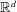in terms of its Fourier transform as a tempered distribution. We conclude by looking at a large class of such measures coming from the cut and project formalism.

]]>
<![CDATA[The Bombieri–Vinogradov Theorem on Higher Rank Groups and its Applications]]>

We study the analogue of the Bombieri–Vinogradov theorem for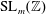Hecke–Maass form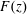. In particular, for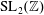holomorphic or Maass Hecke eigenforms, symmetric-square lifts of holomorphic Hecke eigenforms on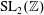, and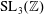Maass Hecke eigenforms under the Ramanujan conjecture, the levels of distribution are all equal to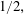which is as strong as the Bombieri–Vinogradov theorem. As an application, we study an automorphic version of Titchmarch’s divisor problem; namely for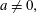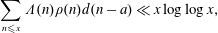where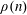are Fourier coefficients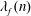of a holomorphic Hecke eigenform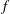foror Fourier coefficients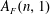of its symmetric-square lift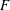. Further, as a consequence, we get an asymptotic formula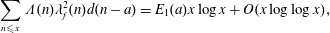where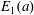is a constant depending on. Moreover, we also consider the asymptotic orthogonality of the Möbius function against the arithmetic function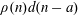.

]]>
<![CDATA[Eigenvalue Optimisation on Flat Tori and Lattice Points in Anisotropically Expanding Domains]]>

This paper is concerned with the maximisation of the-th eigenvalue of the Laplacian amongst flat tori of unit volume in dimension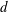asgoes to infinity. We show that in any dimension maximisers exist for any given, but that any sequence of maximisers degenerates asgoes to infinity when the dimension is at most 10. Furthermore, we obtain specific upper and lower bounds for the injectivity radius of any sequence of maximisers. We also prove that flat Klein bottles maximising the-th eigenvalue of the Laplacian exhibit the same behaviour. These results contrast with those obtained recently by Gittins and Larson, stating that sequences of optimal cuboids for either Dirichlet or Neumann boundary conditions converge to the cube no matter the dimension. We obtain these results via Weyl asymptotics with explicit control of the remainder in terms of the injectivity radius. We reduce the problem at hand to counting lattice points inside anisotropically expanding domains, where we generalise methods of Yu. Kordyukov and A. Yakovlev by considering domains that expand at different rates in various directions.

]]>
<![CDATA[Large Irredundant Sets in Operator Algebras]]>

A subset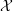of a C*-algebra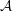is called irredundant if no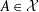belongs to the C*-subalgebra ofgenerated by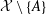. Separable C*-algebras cannot have uncountable irredundant sets and all members of many classes of nonseparable C*-algebras, e.g., infinite dimensional von Neumann algebras have irredundant sets of cardinality continuum.

There exists a considerable literature showing that the question whether every AF commutative nonseparable C*-algebra has an uncountable irredundant set is sensitive to additional set-theoretic axioms, and we investigate here the noncommutative case.

Assuming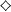(an additional axiom stronger than the continuum hypothesis), we prove that there is an AF C*-subalgebra of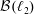of density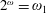with no nonseparable commutative C*-subalgebra and with no uncountable irredundant set. On the other hand we also prove that it is consistent that every discrete collection of operators inof cardinality continuum contains an irredundant subcollection of cardinality continuum.

Other partial results and more open problems are presented.

]]>
<![CDATA[From Steklov to Neumann and Beyond, via Robin: The Szegő Way]]>

The second eigenvalue of the Robin Laplacian is shown to be maximal for the disk among simply-connected planar domains of fixed area when the Robin parameter is scaled by perimeter in the form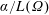, andlies between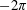and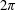. Corollaries include Szegő’s sharp upper bound on the second eigenvalue of the Neumann Laplacian under area normalization, and Weinstock’s inequality for the first nonzero Steklov eigenvalue for simply-connected domains of given perimeter.

The first Robin eigenvalue is maximal, under the same conditions, for the degenerate rectangle. When area normalization on the domain is changed to conformal mapping normalization and the Robin parameter is positive, the maximiser of the first eigenvalue changes back to the disk.

]]>
https://dx.doi.org/10.4153/S0008414X19000154?rft_dat=source%3Ddrssdoi:10.4153/S0008414X19000154From Steklov to Neumann and Beyond, via Robin: The Szegő Way1024104310.4153/S0008414X19000154https://dx.doi.org/10.4153/S0008414X19000154?rft_dat=source%3Ddrss2019-03-07Canadian Journal of Mathematics2019-03-07Freitas, PedroLaugesen, Richard S.
<![CDATA[Heisenberg Modules over Quantum 2-tori are Metrized Quantum Vector Bundles]]>

The modular Gromov–Hausdorff propinquity is a distance on classes of modules endowed with quantum metric information, in the form of a metric form of a connection and a left Hilbert module structure. This paper proves that the family of Heisenberg modules over quantum two tori, when endowed with their canonical connections, form a family of metrized quantum vector bundles, as a first step in proving that Heisenberg modules form a continuous family for the modular Gromov–Hausdorff propinquity.

]]>